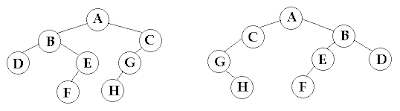Question: Two trees s and t are quasi-isomorphic if s can be transformed into t by swapping left and right children of some of the nodes of s. The values in the nodes are not important in determining quasi-isomorphism, only the shape is important. The trees below are quasi-isomorphic because if the children of the nodes A, B, and G in the tree on the left are swapped, the tree on the right is obtained. Write a method isQuasiIsomorphic that returns true if two trees are quasi-isomorphic.Code:

`  bool quasiisomorphic(struct treenode *treeone, struct treenode *treetwo){    if (!treeone && !treetwo)        return true;     if((!treeone && treetwo) || (treeone && !treetwo))        return false;     return (quasiisomorphic(treeone->left, treetwo->left)        && quasiisomorphic(treeone->right, treetwo->right)        || quasiisomorphic(treeone->right, treetwo->left)        && quasiisomorphic(treeone->left, treetwo->right));}`